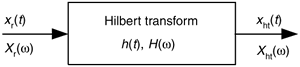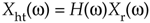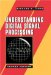# HILBERT TRANSFORM DEFINITION

The Hilbert transform (HT) is a mathematical process performed on a real signal xr(t) yielding a new real signal xht(t), as shown in Figure 9-1.

Figure 9-1. The notation used to define the continuous Hilbert transform.Our goal here is to ensure that xht(t) is a 90o phase-shifted version of xr(t). So, before we carry on, let's make sure we understand the notation used in Figure 9-1. The variables are defined as:

 xr(t) = a real continuous time-domain input signal h(t) = the time impulse response of a Hilbert transformer xht(t) = the HT of xr(t), (xht(t) is also a real time-domain signal) Xr(w) = the Fourier transform of real input xr(t) H(w) = the frequency response (complex) of a Hilbert transformer Xht(w) = the Fourier transform of output xht(t) w = continuous frequency measured in radians/second t = continuous time measured in seconds.

We'll clarify that xht(t) = h(t)*xr(t), where the * symbol means convolution. In addition, we can define the spectrum of xht(t) as Xht(w) = H(w)·Xr(w). (These relationships sure make the HT look like a filter, don't they? We'll cogitate on this notion again later in this chapter.)

Describing how the new xht(t) signal, the HT of xr(t), differs from the original xr(t) is most succinctly done by relating their Fourier transforms, Xr(w) and Xht(w). In words, we can say that all of xht(t)'s positive frequency components are equal to xr(t)'s positive frequency components shifted in phase by –90o. Also, all of xht(t)'s negative frequency components are equal to xr(t)'s negative frequency components shifted in phase by +90o. Mathematically, we recall:where H(w) = –j over the positive frequency range, and H(w) = +j over the negative frequency range. We show the non-zero imaginary part of H(w) in Figure 9-2(a).

Figure 9-2. The complex frequency response of H(w).To fully depict the complex H(w), we show it as floating in a three-dimensional space in Figure 9-2(b). The bold curve is our complex H(w). On the right side is an upright plane on which we can project the imaginary part of H(w). At the bottom of Figure 9-2(b) is a flat plane on which we can project the real part of H(w). In rectangular notation, we say that H(w) = 0 +j1 for negative frequencies and H(w) = 0 –j1 for positive frequencies. (We introduce the three-dimensional axes of Figure 9-2(b) now because we'll be using it to look at other complex frequency-domain functions later in this discussion.)

To show a simple example of a HT, and to reinforce our graphical viewpoint, Figure 9-3(a) shows the three-dimensional time and frequency representations of a real cosine wave cos(wt). Figure 9-3(b) shows the HT of cos(wt) to be the sinewave sin(wt).

Figure 9-3. The Hilbert transform: (a) cos(wt); (b) its transform is sin(wt).The complex spectrum on the right side of Figure 9-3(b) shows how the HT rotates the cosine wave's positive frequency spectral component by –j, and the cosine wave's negative frequency spectral component by +j. You can see on the right side of Figure 9-3 that our definition of the +j multiplication operation is a +90o rotation of a spectral component counterclockwise about the frequency axis. (The length of those spectral components is half the peak amplitude of the original cosine wave.) We're assuming those sinusoids on the left in Figure 9-3 exist for all time, and this allows us to show their spectra as infinitely narrow impulses in the frequency domain.

Now that we have this frequency response of the HT defined, it's reasonable for the beginner to ask: "Why would anyone want a process whose frequency domain response is that weird H(w) in Figure 9-2(b)?"

URL http://proquest.safaribooksonline.com/0131089897/ch09lev1sec1AmazonPrev don't be afraid of buying books NextUnderstanding Digital Signal Processing (2nd Edition) ISBN: 0131089897EAN: 2147483647 Year: 2004Pages: 183 Authors: Richard G. Lyons BUY ON AMAZON Flylib.com © 2008-2020. If you may any questions please contact us: flylib@qtcs.net